×

Search anything:

# Time bounds for selection

#### Algorithms Time ComplexityIn this article, we have discussed the paper titled "Time bounds for selection" submitted by Manuel Blum, Robert W. Floyd, Vaughan Pratt, Ronal L. Rivest, AND Robert E. Tarjan. This paper proposed a new algorithm called PICK for the problem that is stated as "Given an array of n integers, we have to pick the ith smallest number". The algorithm demonstrates to solve in no more than 5.4305 * n comparisons.

# Background

The problem can be traced back to the world of sports and the design of tournament to select first and second best player.The first best player will obviously be the one who won the entire game, however the previous method was denounced where the player who loses the final match was the second best player. Around 1930, this problem came into the realm of algorithmic complexity. It was shown that no more than n + [log(n)]- 2 matches are required, this method conducted a second match for the log(n) (at most) players other than the winner to determine the second best player.

There are a certain notations that we need to understand here.

1. ith of S: S is a set of numbers and ith of S denotes the ith smallest element in S.
2. rank of x: rank of an element in an array can be defined as the number of elements in S, that are lesser than x.
Also, x has to be such that
``````y = rank of x
yth of S has to be x (yth of S = x)
``````

See the below figure for better understanding.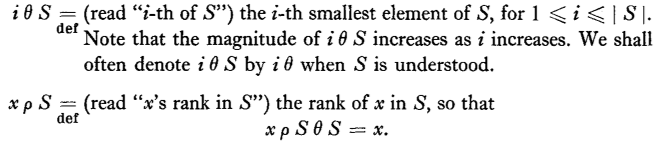The minimum worst-case cost, that is, the number of binary comparisons required, to select ith of S will be denoted by f(i, n), |S|=n, and a new notation is introduced to measure the relative difficulty of computing percentile levels.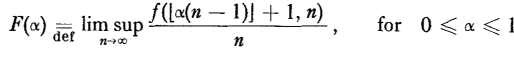# PICK

Pick operates by successively removing subsets of S whose elements are known to be too small or too large to the iΘS, until only ith of S remains. Each subset removed with contain at least one quarter of the remaining elements. PICK will be described in terms of three auxiliary functions b(i,n), c(i,n), d(i,n). In order to avoid confusion we will omit argument lists for these functions.

Let's see the algorithm

1. Select m which belongs to S
a) Arrange S into n/c columns of length c and sort each column
b) Select m such that it is the bth of T, where T contains elements from the set whose size is n/c and are the dth smallest element from each column. Use PICK recursively if n/c>1
2. Compute rank of m
Compare m to every other element x in S for which it is not known whether m>x or m<x.
3. If rank of m is i, then we've got our answer, since m is the ith of S,
if rank of m > i, we can disregard/remove D = set of elements greater than m, and n becomes n-number of elements present in D,
if rank of m < i, then we can disregard/remove D = set of elements lesser than m, and n becomes n-number of elements present in D.
Go back to Step 1.

Pseudo code for above algorithm would look like
PICK(a[], n, i)

1. if(n<1) stop
2. let k=n/c
3. divide a[] into k pairs
4. form another pair with dth smallest element from each pair.
5. m = rank(d)
6. if m=i, halt
else if(m>i) discard set D = {x>=i}, where x belongs to a
else discard set D = {x<=i}
7. repeat recursively till k>1

## Proving that f(n,i) = O(n)

The functions b(), c() and d() decide the time complexity, we need to choose them wisely as an additional sorting is also done.
Let h(c) denote cost of sorting c elements using Ford and Johnson's algorithm. Also known as the merge-insertion sort algorithm is a comparison sorting algorithm that involves fewer comparisons in the worst case as compared to insertion and merge sorts. The algorithm involves three steps, let n be the size of array to be sorted.

1. Split the array into n/2 pairs of two elements and order them pairwise, if n is odd, then the last element isn't paired with any element.
2. The pairs are recursively sorted by their highest value. Again, in case of n being odd, the last element is not considered highest value and left at the end of collection. Now all the highest elements form a list, let's call it main list (a1, a2,...,an/2) and the remaining ones are the pend list(b1, b2.....,bn/2), for any given i bi<=ai.
3. Insert pend elements into the main list, we know that b1<=a1, hence {b1,a1,a2,....,an/2}. Now the remaining elements should be inserted with the constraint that the size of insertion is 2^(n)-1. This process should be repeated till all the elements are inserted.

And the cost of sorting elements is given below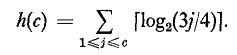Now the cost of 1a previously described is n.h(c)/c. Hence, it's only wise to make c a constant in order for the algorithm to run in linear time.
Let P(n) be the maximum cost for PICK for any i. We can bound the cost of 1b by P(n/c). After 1b a partial order is formed which can be seen in the below figure.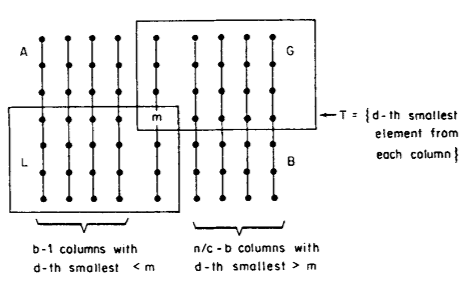Since the recursive call to PICK in step l(b) determines which elements of T are <m, and which are >m, we separate the columns, where every element of box G is clearly greater than m, while every element in box L is less. Therefore, only those elements in quadrants A and B need to be compared to m in step 2.

We can easily show that no elements are removed incorrectly in step 3. if rank of m > i, we can say that m is too large and remove all elements greater than m and vice versa. Also, note that at the least all of L or G is removed.
Therefore

``````P(n) <= n.h(c)/c + P(n/c) + n + P(n - min(|L| , |G|)
The values of b,c,d decide much of the computation cost. To minimise the aboce equation we can assume some values and proceed further.
Let c=21
d=11
b=n/2*c =>n/42
By substituting the above values in the equation
P(n) <= 66n/21 + P(n/21) + n + P(31n/42)
P(n) <= 58*n/3 = 19.6n
``````

The basis for the induction is that since h(n) < 19n for n < l0 5, any small case can be handled by sorting. PICK runs in linear time because a significant fraction of S is discarded on each pass, at a cost proportional to the number of elements discarded on each step. Note that we must have c >= 5 for PICK to run in linear time.

# Values of b, c, d

1. A reasonable choice for these functions can help the algorithm achieve a linear time. From the above calculations it has been proven that c must be constant, since the cost of sorting depends on it. Also, it must be greater than or equal to 5.
2. We have defined P(n) as maximum cost of PICK for any i, therefore we chose c and d to be constants and b as a function of c, as b decides the value of m(recall 1b) and therefore it would make sense to choose b in terms of c.

## Improvements to PICK

Two modifications to PICK are described,

1. PICK1 which yields best overall bound for F(α)
2. PICK2 which is more efficient for i in the ranges of i < ßn or i > (1-ß)n for ß = 0.203688.

PICK1 differs by PICK in

1. The elements of S are sorted into columns only once, after which those columns broken by the discard operation are restored to full length by a (new) merge step at the end of each pass.
2. The partitioning step is modified so that the number of comparisons used is a linear function of the number of elements eventually discarded.
3. The discard operation breaks no more than half the columns on each pass, allowing the other modifications to work well.
4. The sorting step implicit in the recursive call to select m is partially replaced by a merge step for the second and subsequent iterations since (3) implies that 1/2 of the set T operated on at pass j were also in the recursive call at pass j- 1.

The procedure of PICK1 is relatively lengthy. The optimized algorithm is full of red tape, in principle, for any particular n could be expanded into a decision tree without red-tape computation.

PICK2

By analysis of PICK2, which is essentially the same as PICK with the functions b(i, n), c(i, n), and d(i, n) chosen to be i, 2, and 1, respectively, and with the partitioning step eliminated.

Time Complexity

The main result i.e time complexity of PICK , f(i,n) = O(n). However, this has been tuned up to provide tighter results. If you recall the definition of F(α) to measure relative difficulty of computing percentile levels. After the changes made to PICK, we get three expressions here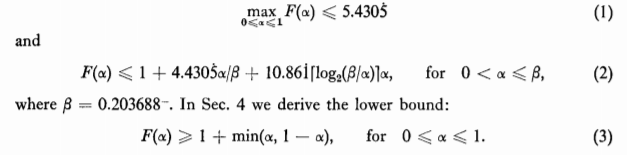There is no evidence to suggest that any of the inequalities (1) - (3) is the best possible. In fact, the authors surmise that they can be improved considerably.

# Conclusion

The most important result of this paper is that selection can be performed in linear time, in the worst case. No more than 5.4305n comparisons are required to select the ith smallest of n numbers, for any i, 1<= i <= n. This bound can be improved when i is near the ends of its range, i.e when i get larger.

With this article at OpenGenus, you must have a strong sense of time complexity analysis of algorithmic problems and understand how selecting any element at a specific order takes only linear time.#### K. Sai Drishya

Love music; Student at Gandhi Institute of Technology and Management (GITAM); Intern at OpenGenus

Time bounds for selection